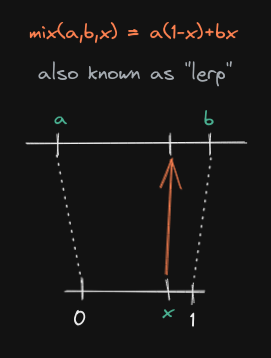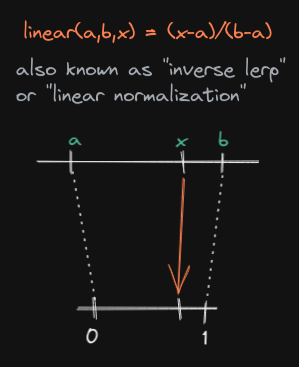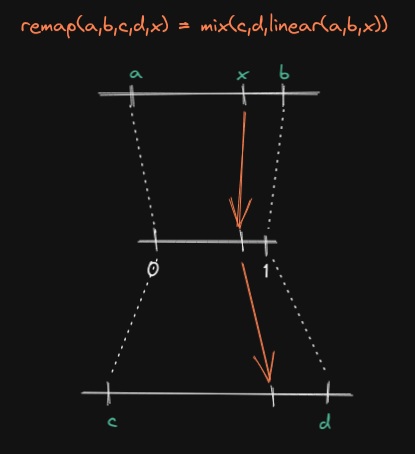# The most useful math formulas

2021-06-09

These last years, in the context of working on a graphic rendering engine, I've been confronted to many engineering problems involving mathematics: basic geometry, colorimetry, curves, animations, video, etc. In all these situations, I needed at some point to formalize a "space conversion" of some sort.

My mathematical background is extremely fragile, but coming up with some very basic tooling for these operations helped me building up my mental models so much faster. These formulas were by far the most impactful in my daily struggle with maths.

## Formula 1: from normalized input to whatever output

Anytime you try to abstract a concept mathematically, you're usually working within the `[0;1]` range, the "purest" data form representation. This is often referred as the "normalized form". When we have a value in this range, we can map it to any range using a `mix()` function, also known as `lerp()`:The function is known to have 2 forms, both having their use-case:

1. `mix(a,b,x) = a*(1-x) + bx`
2. `mix(a,b,x) = x*(b-a) + a`

The first one is numerically stable, meaning that's the form we usually want to use in the code, because it will lead to less floating point inaccuracies.

But the 2nd one is also useful because it does not repeat the `x` input. I find it handy to work with when doing substitution in basic algebra. It also notably follows the well-known polynomial form. Finally, It's also easier to reason with: `b-a` represents the total distance ("end minus start"), `+ a` represents the offsetting, and `x` represents the "amount" of the distance.

Out of all the formulas in the article, this is the most popular one. It's often referred as the "linear interpolation", but I don't like this naming because it directly conflicts with the 2nd formula doing the exact opposite.

It's so popular that GLSL does have it builtin. The wide variety of applications makes it impossible to make an exhaustive list, but if I had to pick one example it would be the mix of two colors when doing a gradient: if you want a certain percentage of a color, and the remaining of another color, this is what you will use (let's ignore gamma correction for now, alright?).

## Formula 2: from whatever input to normalized output

This 2nd formula is also a "linear interpolation", except it's the exact opposite: we're going from an arbitrary range to a normalized one. The use of the same term to refer to both functions was a huge source of confusion for me in the past. Since this function has no official name, I will simply refer to it as `linear(a,b,x) = (x-a)/(b-a)`, or sometimes `linear_norm()`. Some people also like to call it "inverse lerp":This complements `mix()` in such an elegant way, it's a real shame it has no "official" name. The usefulness of this function is basically to provide a way of normalizing a value from any space/range. Said differently, this function is a way of getting a standard and re-usable answer to the question "where is `x` located between `a` and `b`?"

## Formula 3: From whatever input to whatever output

Now with the help of `mix()` and `linear()` we can now go from one range to any other: `remap(a,b,c,d,x) = mix(c,d,linear(a,b,x))`.The logic is pretty straightforward: we have a value in the range `[a;b]`, which we first normalize with `linear()`, then we directly use that result to signify where we want to land on `[c;d]` using `mix()`.

If you develop the expression, you will get the following useful polynomial form: `remap(a,b,c,d,x) = x*(d-c)/(b-a) + c-a*(d-c)/(b-a)`.

## Step and non-linear variants

You may see `linearstep()` in the wild, which is simply `linear()` with a `[0;1]` clamping: `linearstep(a,b,x) = saturate(linear(a,b,x))` (which is useful in case `x` is outside `[a;b]`).

If you've worked with GLSL, you might have also heard of `smoothstep()`. Instead of being linear, it follows a curve, but in its implementation it's using `linearstep()` (hence the "step"), then bends the results ("smooth") using a Hermite polynomial:

`smoothstep(a,b,x) = 3t²-2t³` with `t = linearstep(a,b,x)`

## Bonus: relationship between these formulas

If like me you're wondering why `mix()` and `linear()` look so different even though their concept is so close, following is a demonstration for going from one formula to another with basic algebra:

### mix → linear

``````y = a*(1-x)+bx
y = a - ax + bx
y = a + bx - ax
y-a = x * (b-a)
x = (y-a)/(b-a)
``````

### linear → mix

``````x = (y-a)/(b-a)
x*(b-a) = y-a
bx - ax + a = y
y = a*(1-x)+bx
``````

## Conclusion

I'm sure many of the people reading this will sigh at such elementary maths, but nowadays, not a week passes without me looking at the visual representation of `mix()` and `linear()` when building various mental models for the concepts I work with. I'm hoping this will be useful to other people.

For updates and more frequent content you can follow me on Mastodon. Feel also free to subscribe to the RSS in order to be notified of new write-ups. It is also usually possible to reach me through other means (check the footer below). Finally, discussions on some of the articles can sometimes be found on HackerNews, Lobste.rs and Reddit.